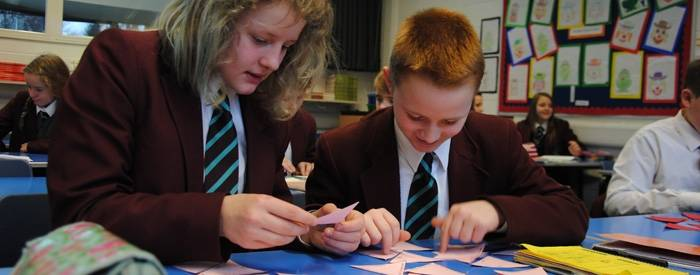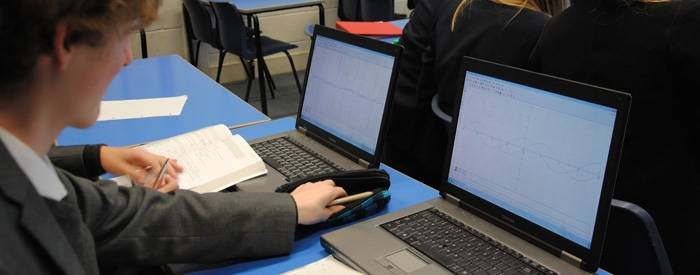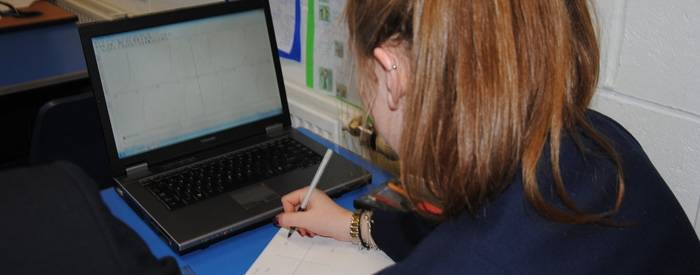•••Mathematics

### Key Stage 3   |    Key Stage 4   |    Key Stage 5

Maths is important for all members of modern society. Mathematical thinking and the use of mathematical techniques is part of the essential equipment that all students need to enter the world of work and decision making.

We believe that Maths should also be a stimulating and enjoyable experience for all of our students, helping them to learn the basic skills and to develop the ability to solve problems in a creative way.

We strive to create an atmosphere where students are challenged and encouraged to do their best.

### NATIONAL CURRICULUM

The Ecclesbourne School follows the 3 Year National Curriculum

The national curriculum for mathematics aims to ensure that all pupils:

• become fluent in the fundamentals of mathematics, including through varied and frequent practice with increasingly complex problems over time, so that pupils develop conceptual understanding and the ability to recall and apply knowledge rapidly and accurately.
• reason mathematicallyby following a line of enquiry, conjecturing relationships and generalisations, and developing an argument, justification or proof using mathematical language
• can solve problems by applying their mathematics to a variety of routine and non-routine problems with increasing sophistication, including breaking down problems into a series of simpler steps and persevering in seeking solutions.

### Curriculum Intent

Mathematics is a creative and highly inter-connected discipline that has been developed over centuries, providing the solution to some of history’s most intriguing problems. It is essential to everyday life, critical to science, technology and engineering, and necessary for financial literacy and most forms of employment. A high-quality mathematics education therefore provides a foundation for understanding the world, the ability to reason mathematically, an appreciation of the beauty and power of mathematics, and a sense of enjoyment and curiosity about the subject.

### Course Assessment

9 Students can solve quadratic inequalities in one variable. Students can represent the solution set of inequalities on a number line, using set notation and on a graph. Students can sketch translations and reflections of a given function, and can find the equation of a tangent to a circle at a given point. Students can interpret the succession of two functions as a ‘composite function’. Students use circle theorums to prove related results.
8 Students can solve quadratic equations by completing the square. Students can solve two simultaneous equations in two variables (linear/quadratic) algebraically, and can find approximate solutions to equations numerically using iteration. Students can recognise, sketch and interpret the trigometric functions y=sinx, y=cosx and y=tanx for angles of any size. Students can calculate or estimate gradients of graphs and areas under graphs (including quadratic and other non-linear graphs) and interpret results in cases such as distance-time graphs. Students can argue mathematically to show algebraic expressions are equivalent, and use algebra to support and construct proofs.
7 Students can simplify surd expressions involving squares, and can calculate upper and lower bounds. Students can factorise quadratic expressions, and solve quadratic equations using the quadratic formula. Students can use vectors to construct geometric arguments and proofs, and can understand 3D pythagoras. Students know and can apply the sine rule and cosine rule to find unknown lengths and angles. Students can construct and interpret diagrams for grouped discrete data and continuous data i.e. histograms with equal and unequal class intervals and cumulative frequency graphs.
6 Students can change recurring decimals into their corresponding fractions and vice versa. Students can calculate fractional indices. Students can simplify the laws of indices, and solve quadratic equations. Students can find approximate solutions using a graph. Students can to identify perpendicular lines. Students can deduce expressions to calculate the nth tetm of a quadratic sequence. Students can solve direct and inverse proportion problems. Students can apply the standard circle theorums concerning angles, radii, tangents and chords. Students can apply the concepts of congruence and similarity, including the relationships between areas and volumes in similar figures. Students can calculate and interpret conditional probabilities through representation using expected frequencies with a variety of tables and graphs.
5 Students use inequality notation and can interpret fractions and percentages as operators. Students can solve linear equations in one unknown algebraically, and solve two simultaneous equations in two variables algebraically. Students can solve linear inequalities in two variables. Students can find the equation of the line through two given points or through one point with a given gradient. Students can rearrange formulae to change the subject and can interpret the gradient of a straight line graph as a rate of change. Students can solve original value problems. Students can use the basic congruence criteria for triangles and know the formulae for the trigonometric ratios. Students can calculate surface area and volumes of spheres, pyramids, cones and composite solids. Students can calculate probabilty of events and can find the median from cumulative frequency graphs.
4 Students can use the concepts and vocabulary of prime factorisation, and check calculations using approximations. Students can round numbers to an approximate degree of accuracy, and can calculate with integer indices. Students can solve linear equations in one unknown algebraically. Students can find approximate solutions using a graph. Students can recognise, sketch and interpret graphs of linear functions, and can identify parallel lines. Students can divide a quanity into two parts in a given ratio, and can solve problems using simple percentages. Students understand the sum of angles within a triangle and are familiar with Pythagoras’ theorem. Students can find the mean, median and modal averages from a data set, and draw estimated lines of best fit.
3 Students can apply the four operations, and can round numbers to a degree of accuracy. Students can use positive integer powers and associated real roots. Students can substitute numerical values into formulae and expressions. Students can multiply a single term over a bracket. Students can plot simple graphs with given co-ordinates. Students can solve problems using percentages. Students can describe translations as 2D vectors, and can apply formulae to calculate volumes, and circle geometry. Students can compare data using averages and range.
2 Students can order decimals and fractions, and collect like terms. Students can express one quantity as a percentage of another. Students can apply properties of angles at a point, and on a straight line, which being able to measure angles to calculate the area of basic shapes. Students can find the mean from a list of data, and make comparisons using charts.
1 Students can order positive and negative integers. Students can define a percentage as ‘number of parts per hundred’. Students can measure line segments, and calculate the area of a triangle. Students can apply averages to a list of data, and draw and interpret line graphs, bar charts and pictograms. Students can make lists and tally charts.

Contact: Mrs M. Weighill